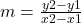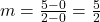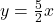## What is the equation of the line through the origin and (2,5)? A. y = 5/2x B. y = 2/5x C. y= 2x D. y = 5x

Question

What is the equation of the line through the origin and (2,5)?
A. y = 5/2x
B. y = 2/5x
C. y= 2x
D. y = 5x

in progress 0
6 months 2021-08-03T14:15:54+00:00 1 Answers 5 views 0

A

Step-by-step explanation:

We can just use the slope equation here.The two given points are (0,0) and (2,5)Since the line intersects the y-axis at the origin, the y-intercept is 0.

So, our slope-intercept equation is.

Hope this helps!